# RD Sharma Class 8 Solutions Chapter 25 Data Handling (Pictorial Representation of Data as Pie Charts or Circle Graphs) Ex 25.1

In this chapter, we provide RD Sharma Class 8 Solutions Chapter 25 Data Handling Ex 25.1 for English medium students, Which will very helpful for every student in their exams. Students can download the latest RD Sharma Class 8 Solutions Chapter 25 Data Handling Ex 25.1 pdf, free RD Sharma Class 8 Solutions Chapter 25 Data Handling Ex 25.1 book pdf download. Now you will get step by step solution to each question.

### RD Sharma Class 8 Solutions Chapter 25 Data Handling Ex 25.1Download PDF

Question 1.
The number of hours, spent by a school boy on different activities in a working day, is given below:Present the information in the form of a pie-chart .
Solution:
Total = 24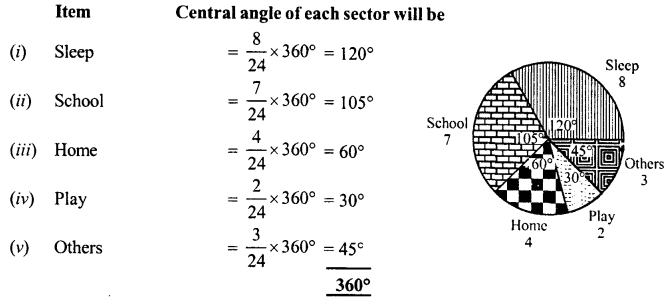Now we draw a circle and divide it in the sectors having above central angles as shown in the figure.

Question 2.
Employees of a company have been categorized according to their religions as given below: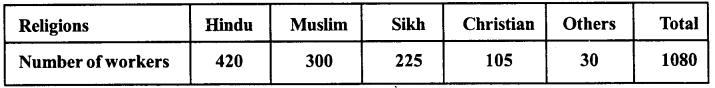Draw a pie-chart to represent the above information.
Solution:
Total =1080Now draw a circle and divided it into sectors having the above central angles as shown in the figure.

Question 3.
In one day the sales (in rupees) of different items of a baker’s shop are given below :Draw pie-chart representing the above sales.
Solution:
Total = 480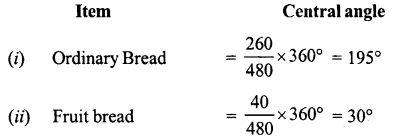Now draw a circle and divide it into sectors having the above central angles as shown in the figure.

Question 4.
The following data shows the expenditure of a person on different items during a month. Represent the data by a pie-chart.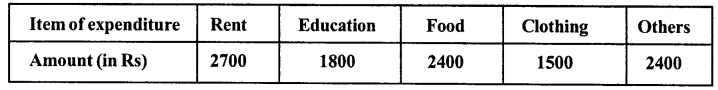Solution:
Total = 10800Now we draw a circle and divide it into sector having the above central angles as shown in figure.

Question 5.
The percentages of various categories of workers in a state are given in the following table: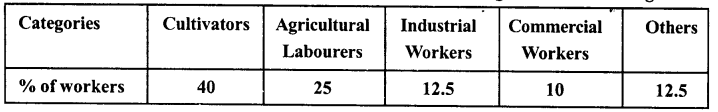Present the information in the form of a pie-chart.
Solution:
Total = 100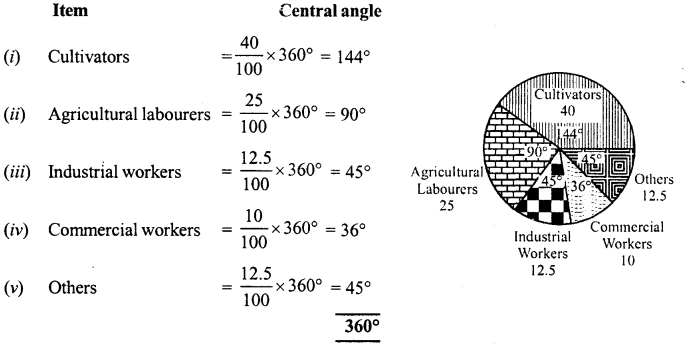https://googleads.g.doubleclick.net/pagead/ads?client=ca-pub-7601472013083661&output=html&h=280&adk=2523109437&adf=980553861&pi=t.aa~a.1381849204~i.18~rp.4&w=750&fwrn=4&fwrnh=100&lmt=1618318950&num_ads=1&rafmt=1&armr=3&sem=mc&pwprc=1894297687&psa=1&ad_type=text_image&format=750×280&url=https%3A%2F%2Fwww.learninsta.com%2Frd-sharma-class-8-solutions-chapter-25-data-handling-iii-ex-25-1%2F&flash=0&fwr=0&pra=3&rh=188&rw=750&rpe=1&resp_fmts=3&wgl=1&fa=27&adsid=ChEI8ILVgwYQgsj-ysyC6vjoARI9AH0NTrfsL400AnizXAqcC1B92sd9a6u_Kf0nPtEMy1DXHbcR-6PvWMg0vNfRjHh1WrSesJ-pEXeWwu5edw&uach=WyJXaW5kb3dzIiwiMTAuMCIsIng4NiIsIiIsIjg5LjAuNDM4OS4xMTQiLFtdXQ..&dt=1618318852269&bpp=1&bdt=4336&idt=1&shv=r20210407&cbv=r20190131&ptt=9&saldr=aa&abxe=1&cookie=ID%3D61b94a43110f009c%3AT%3D1618318853%3AS%3DALNI_MYUKHMK2TEVD7DDPPNRIRSytFnkpw&prev_fmts=0x0%2C750x280%2C1519x763%2C750x280&nras=4&correlator=6305266221728&frm=20&pv=1&ga_vid=133250821.1618207030&ga_sid=1618318851&ga_hid=2050051377&ga_fc=0&u_tz=330&u_his=1&u_java=0&u_h=864&u_w=1536&u_ah=834&u_aw=1536&u_cd=24&u_nplug=3&u_nmime=4&adx=190&ady=4481&biw=1519&bih=763&scr_x=0&scr_y=1474&eid=31060711%2C44740079&oid=3&pvsid=1374720213287893&pem=718&ref=https%3A%2F%2Fwww.learninsta.com%2Frd-sharma-class-8-solutions%2F&eae=0&fc=1408&brdim=0%2C0%2C0%2C0%2C1536%2C0%2C1536%2C834%2C1536%2C763&vis=1&rsz=%7C%7Cs%7C&abl=NS&fu=128&bc=31&ifi=4&uci=a!4&btvi=3&fsb=1&xpc=WOks09BXzc&p=https%3A//www.learninsta.com&dtd=97756

Now, we draw a circle and divide it into sectors having the above central angles as shown in the figure.

Question 6.
The following table shows the expenditure incurred by a publisher in publishing a book:Present the above data in the form of a pie-chart.
Solution:
Total = 100% = 100Now, we draw a circle and divide it into sectors having the above central angles as shown in the figure

Question 7.
Percentage of the different products of a village in a particular district are given below. Draw a pie-chart representing this information.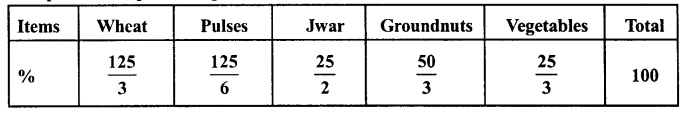Solution:
Total = 100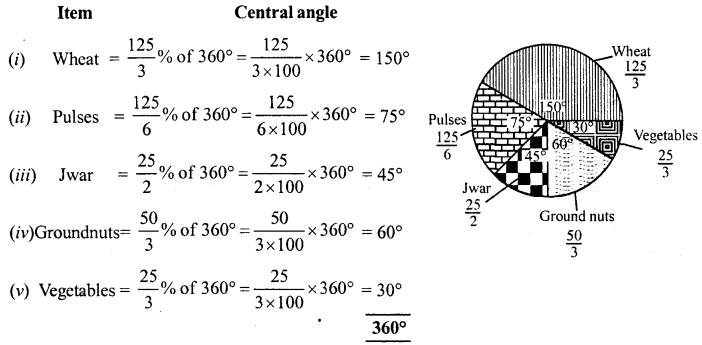Now, we draw a circle and divided it into sectors having the above central angles as shown in the figure.

Question 8.
Draw a pie-diagram for the following data of expenditure pattern in a family.Solution:
Total =100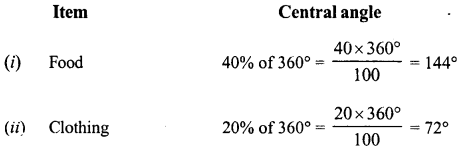Now we draw a circle and divide it into sectors having the above central angles as shown in the figure.

Question 9.
Draw a pie-diagram of the areas of continents cf the world given in the following table :Solution:
Total = 133.3Now, we draw a circle and divide it into sectors having the above given central angles as shown in the figure.

Question 10.
The following data gives the amount spent of the construction of a house. Draw a pie diagram.Solution:
Total = 300 (in thousands)Now, we draw a circle and divide it into sectors having the above given central angles as shown in the figure.

Question 11.
The following table shows how a student spends his pocket money during the course of a month. Represent it by a pie-diagram.Solution:
Total expenditure = 100Now, we draw a circle and divide it into sectors having the above given central angles as shown in the figure.

Question 12.
Represent the following data by a pie-diagram :Solution:
1. For family A
Total = 10000Now, we draw a circle and divide it in sectors having the above central angles as shown in the figure.
(2) For family B
Total = 11680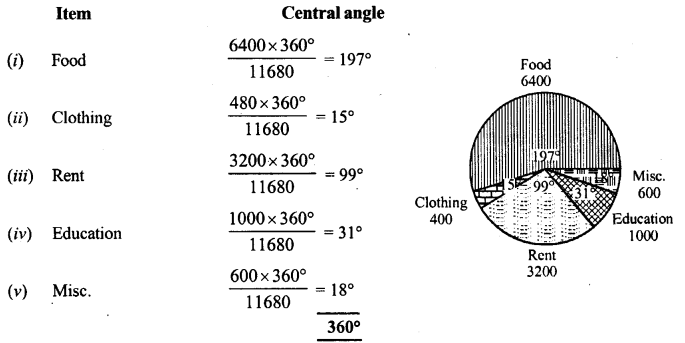Now, we draw a circle and divide it into sectors having the above central angles as shown in the figure.

Question 13.
Following data gives the break up of the cost of production of a book: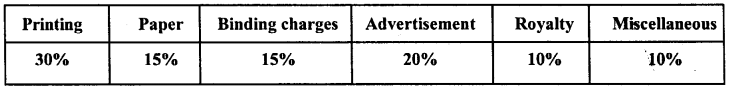Draw a pie – diagram depicting the above information.
Solution:
Total = 100Now, we draw a circle and divide it into sectors of above central angles as shown in the figure.

Question 14.
Represent the following data with the help of pie-diagram.Solution:
Total = 6000 tons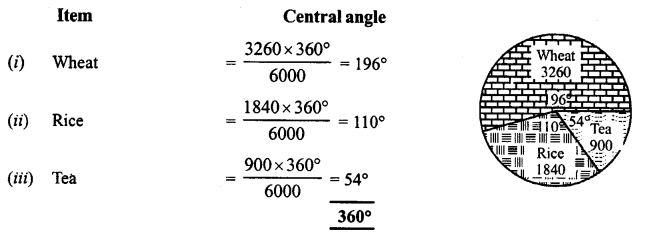Now, we draw a circle and divide it into sectors having the above central angles as shown in the figure.

Question 15.
Draw a pie-diagram representing the relative frequencies (expressed as percentage) of the eight classes as given below :
12.6,18.2,17.5,20.3,2.8,4.2,9.8,14.7
Solution:
Total =100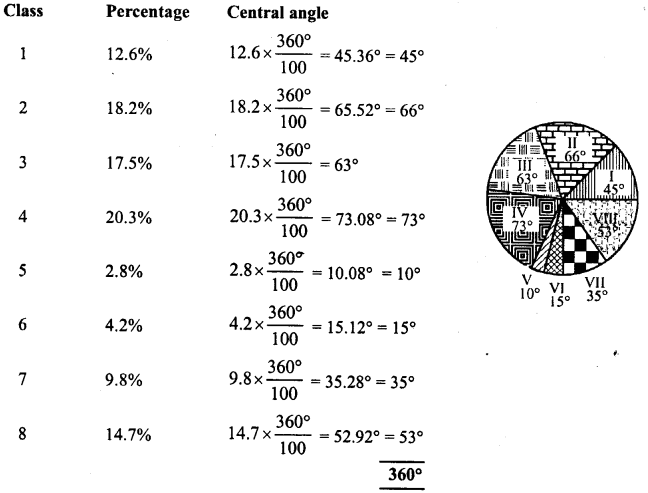Now, we draw a circle and divide it into sectors having the above central angles as shown in the figure given.

Question 16.
Following is the break up of the expenditure of a family on different items of consumption :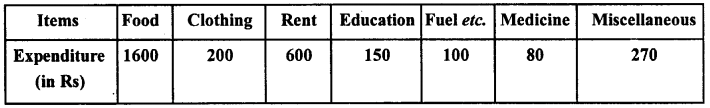Draw a a pie – diagram to represent the above data.
Solution:
Total = Rs. 3000Now, we draw a circle and divide it into sectors having the above central angles as shown in the figure.

Question 17.
Draw a pie-diagram for the following data of the investment pattern in five year plan :Solution:
Total = 100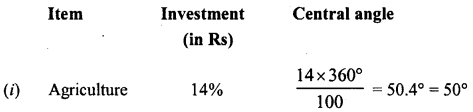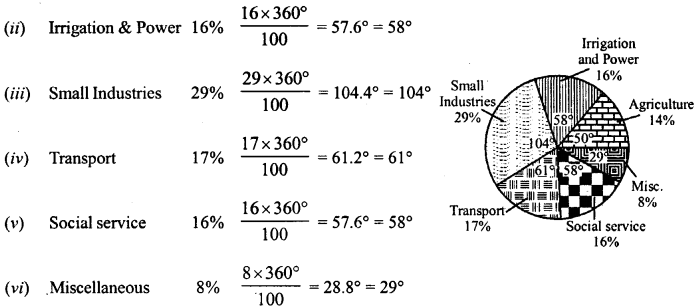All Chapter RD Sharma Solutions For Class 8 Maths

—————————————————————————–

All Subject NCERT Exemplar Problems Solutions For Class 8

All Subject NCERT Solutions For Class 8

*************************************************

I think you got complete solutions for this chapter. If You have any queries regarding this chapter, please comment on the below section our subject teacher will answer you. We tried our best to give complete solutions so you got good marks in your exam.

If these solutions have helped you, you can also share rdsharmasolutions.in to your friends.